# Lesson 4 Three Phase Sources and Loads ET

• Slides: 25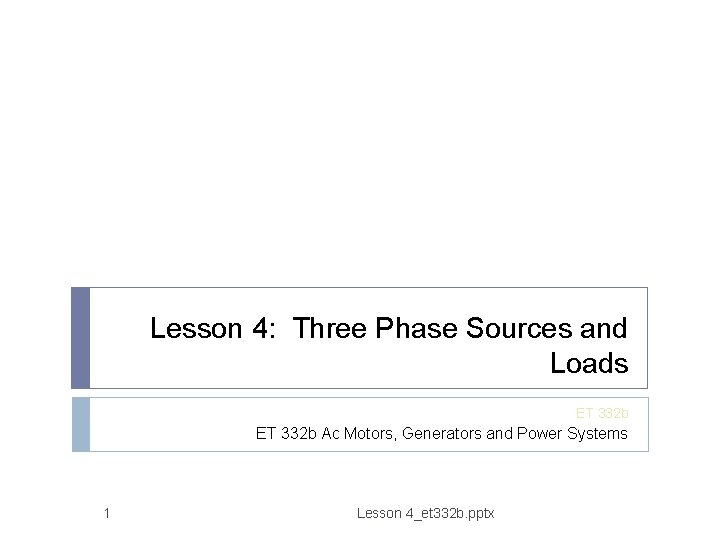Lesson 4: Three Phase Sources and Loads ET 332 b Ac Motors, Generators and Power Systems 1 Lesson 4_et 332 b. pptx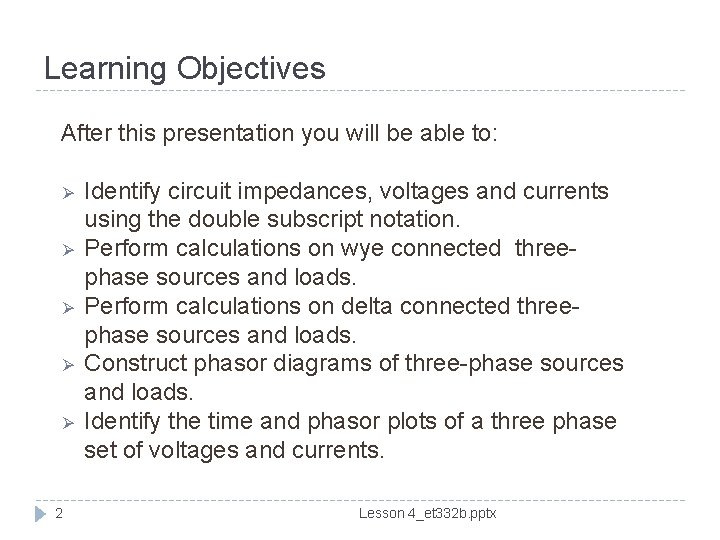Learning Objectives After this presentation you will be able to: Ø Ø Ø 2 Identify circuit impedances, voltages and currents using the double subscript notation. Perform calculations on wye connected threephase sources and loads. Perform calculations on delta connected threephase sources and loads. Construct phasor diagrams of three-phase sources and loads. Identify the time and phasor plots of a three phase set of voltages and currents. Lesson 4_et 332 b. pptxDouble Subscript Notation Sources and voltage drops are defined by the terminal letter. Voltage drop and polarity defined by order of subscripts b a + + Vbn Van - n n Voltages considered positive if first node subscript is higher potential than second node subscript 3 Lesson 4_et 332 b. pptx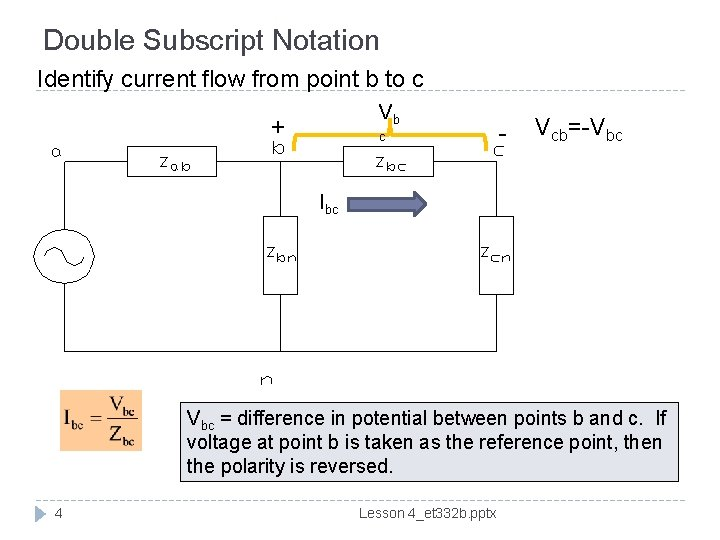Double Subscript Notation Identify current flow from point b to c Vb + c - Vcb=-Vbc Ibc Vbc = difference in potential between points b and c. If voltage at point b is taken as the reference point, then the polarity is reversed. 4 Lesson 4_et 332 b. pptx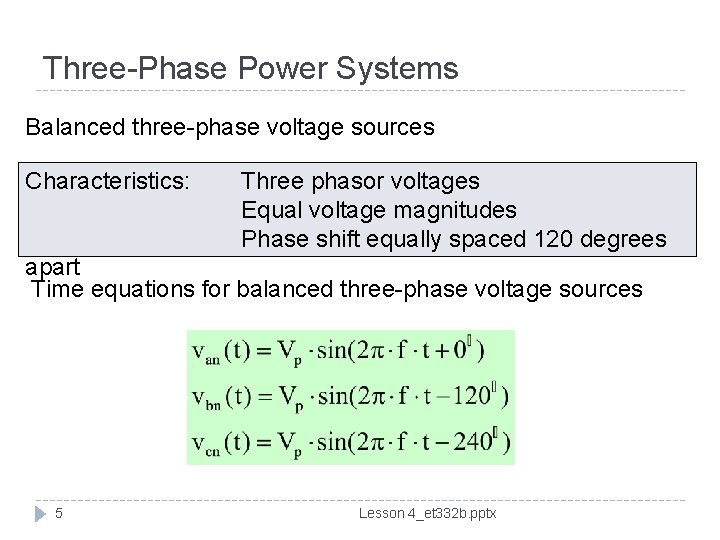Three-Phase Power Systems Balanced three-phase voltage sources Characteristics: Three phasor voltages Equal voltage magnitudes Phase shift equally spaced 120 degrees apart Time equations for balanced three-phase voltage sources 5 Lesson 4_et 332 b. pptx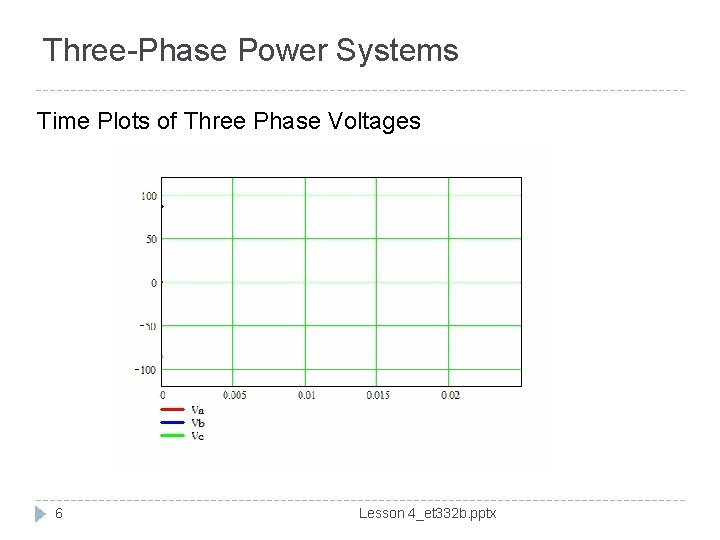Three-Phase Power Systems Time Plots of Three Phase Voltages 6 Lesson 4_et 332 b. pptx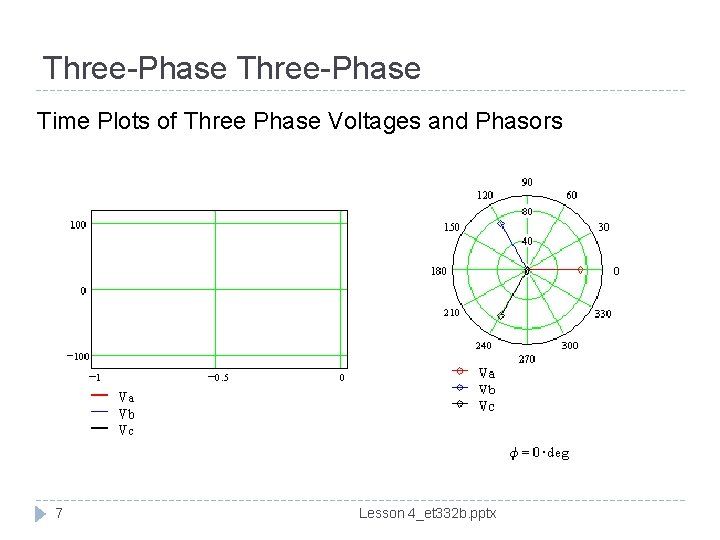Three-Phase Time Plots of Three Phase Voltages and Phasors 7 Lesson 4_et 332 b. pptxThree-Phase Source Connections In wye connection: Wye – Connected, three-phase 3 f sources + Vcn - c n b + - Ic IL=Ip + Vca Van a n - + Ia Where: IL = line current Ip= phase current Vbc + Vab Vbn Ib + Determine the relationship between the magnitude and phase shift of each source voltage and the current and voltage at the terminals of the connection 8 Lesson 4_et 332 b. pptxWye Connected Sources Voltage relationships Line-to-line voltage phasors In balanced systems Vca Perform phasor subtraction to find the values 9 Lesson 4_et 332 b. pptx Vcn -Vbn Vab Van -Vcn Vbc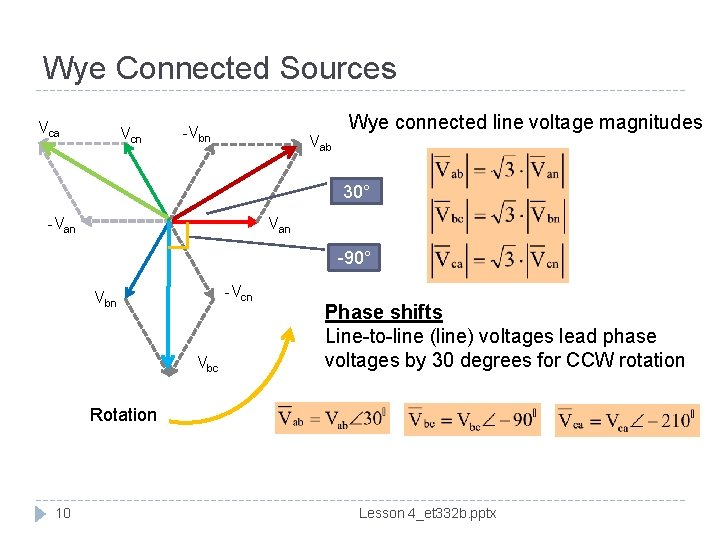Wye Connected Sources Vca Vcn -Vbn Vab Wye connected line voltage magnitudes 30° Van -90° -Vcn Vbc Phase shifts Line-to-line (line) voltages lead phase voltages by 30 degrees for CCW rotation Rotation 10 Lesson 4_et 332 b. pptx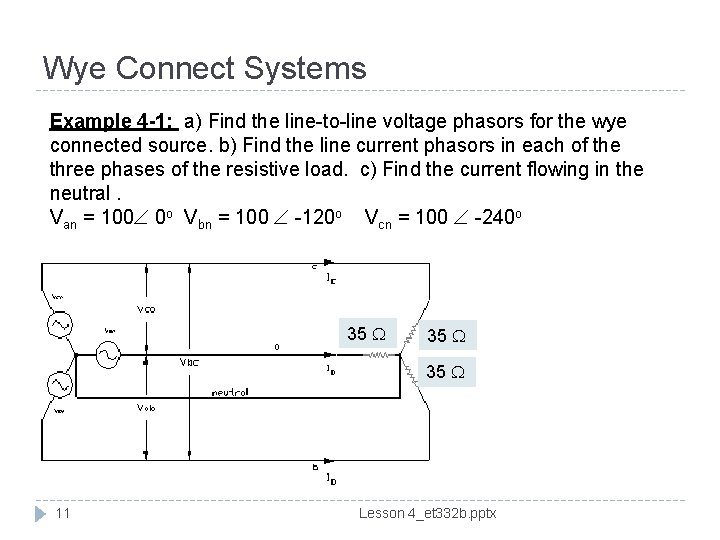Wye Connect Systems Example 4 -1: a) Find the line-to-line voltage phasors for the wye connected source. b) Find the line current phasors in each of the three phases of the resistive load. c) Find the current flowing in the neutral. Van = 100 0 o Vbn = 100 -120 o Vcn = 100 -240 o 35 35 11 Lesson 4_et 332 b. pptxExample 4 -1 Solution (1) Balanced 3 f systems can analyzed using a single phase to neutral and the other quantities determined by the appropriate phase shifts. a) For this sequence of phasors, line-to-line voltages lead phase voltages by 30 degrees Ans 12 Lesson 4_et 332 b. pptx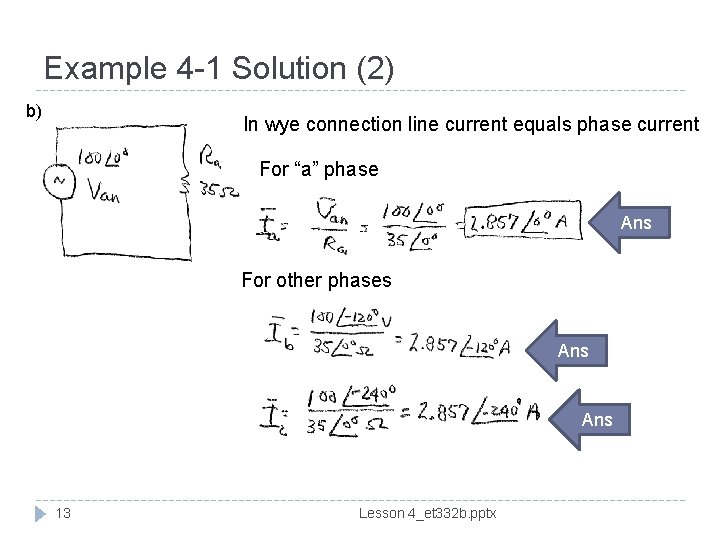Example 4 -1 Solution (2) b) In wye connection line current equals phase current For “a” phase Ans For other phases Ans 13 Lesson 4_et 332 b. pptxExample 4 -1 Solution (3) c) To find the neutral current, sum the currents at the load neutral point Ic Neutral point Ia Convert to rectangular form to add Ib In balanced 3 f system, neutral conductor carries no current. It is used for safety and to handle unbalances 14 Lesson 4_et 332 b. pptxExample 4 -1 Solution (4) Phasor diagram All line-to-line voltages lead the phase voltages by 30 degrees. For resistive load, the phase current is in phase with Vpn, the phase-to-neutral voltage. 15 Lesson 4_et 332 b. pptxDelta Connected Sources Phase voltages are equal to lineto-line voltages in delta connections Vp Ibc VLL Where: VLL = line-to-line voltage Vp = phase voltage So To find relationship between phase and line currents, perform KCL at every corner node 16 Lesson 4_et 332 b. pptx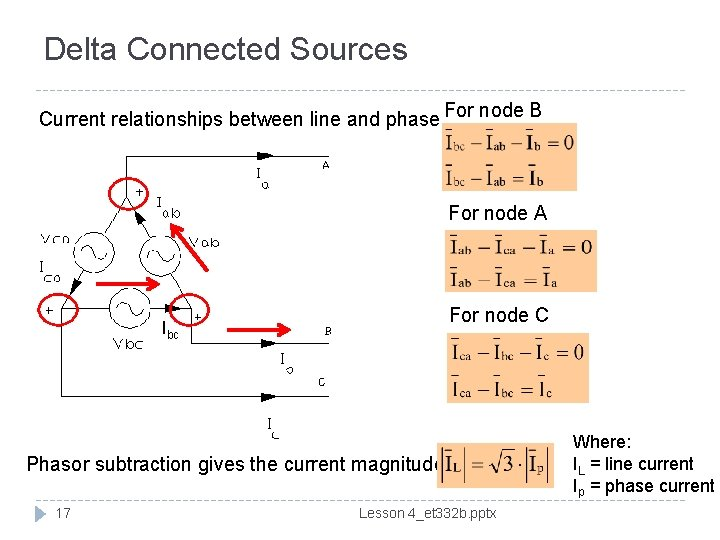Delta Connected Sources Current relationships between line and phase For node B For node A For node C Ibc Phasor subtraction gives the current magnitude 17 Lesson 4_et 332 b. pptx Where: IL = line current Ip = phase currentDelta Connected Sources Phasor diagram of delta currents Line current phasors lag phase currents by 30 degrees in balanced delta connection Rotation -30° Above hold for all phases with Ip as reference phasor Phase rotation is ABC in this case 18 Lesson 4_et 332 b. pptxDelta Connection Example 4 -2: For the delta connected load shown, a) find the phasor values of phase and line currents for the circuit. : b) draw a phasor diagram of the computed currents and given voltages R = 15 ohms in each phase. Vab = 240 0 o Vbc = 240 120 o Vca = 240 o 240 -240 o 240 -120 o 19 Lesson 4_et 332 b. pptx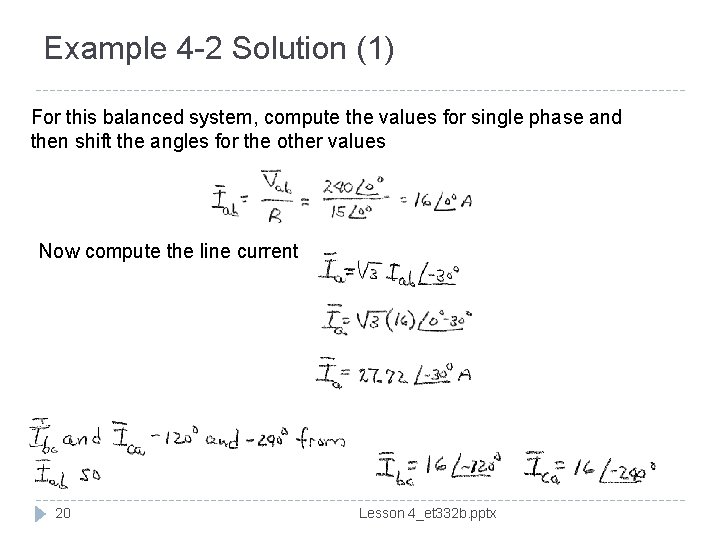Example 4 -2 Solution (1) For this balanced system, compute the values for single phase and then shift the angles for the other values Now compute the line current 20 Lesson 4_et 332 b. pptx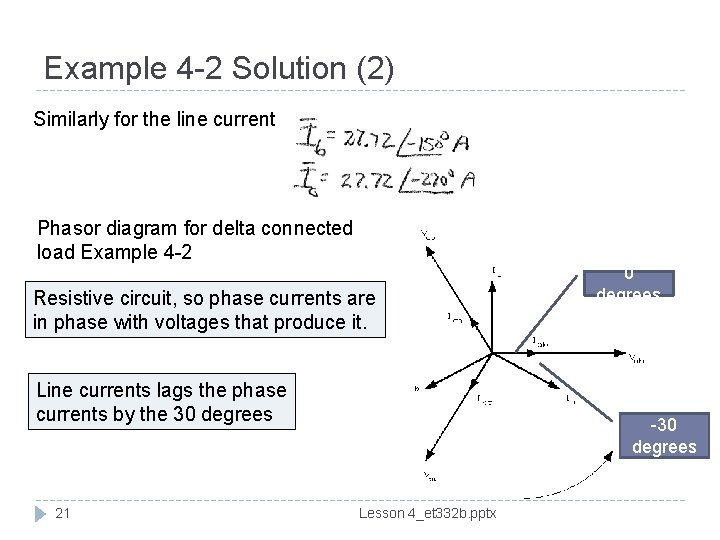Example 4 -2 Solution (2) Similarly for the line current Phasor diagram for delta connected load Example 4 -2 Resistive circuit, so phase currents are in phase with voltages that produce it. Line currents lags the phase currents by the 30 degrees 21 0 degrees -30 degrees Lesson 4_et 332 b. pptx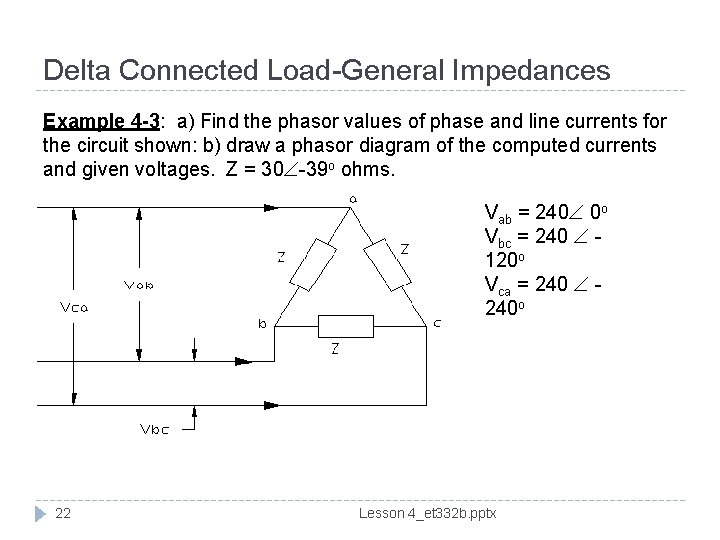Delta Connected Load-General Impedances Example 4 -3: a) Find the phasor values of phase and line currents for the circuit shown: b) draw a phasor diagram of the computed currents and given voltages. Z = 30 -39 o ohms. Vab = 240 0 o Vbc = 240 120 o Vca = 240 o 22 Lesson 4_et 332 b. pptx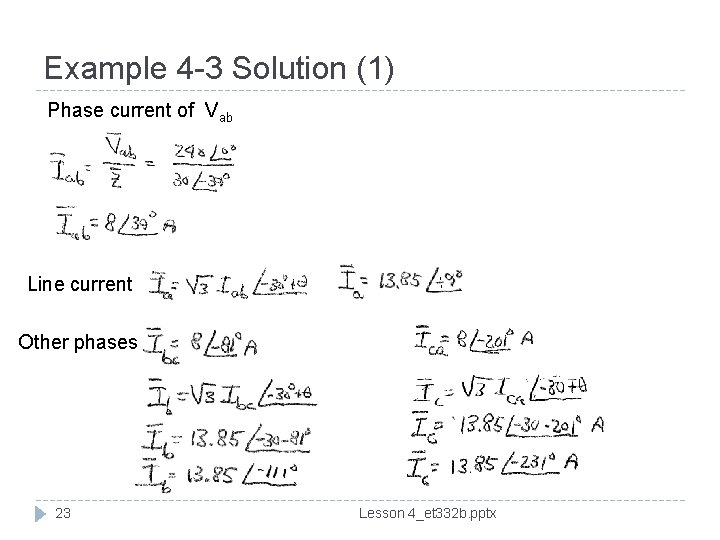Example 4 -3 Solution (1) Phase current of Vab Line current Other phases 23 Lesson 4_et 332 b. pptx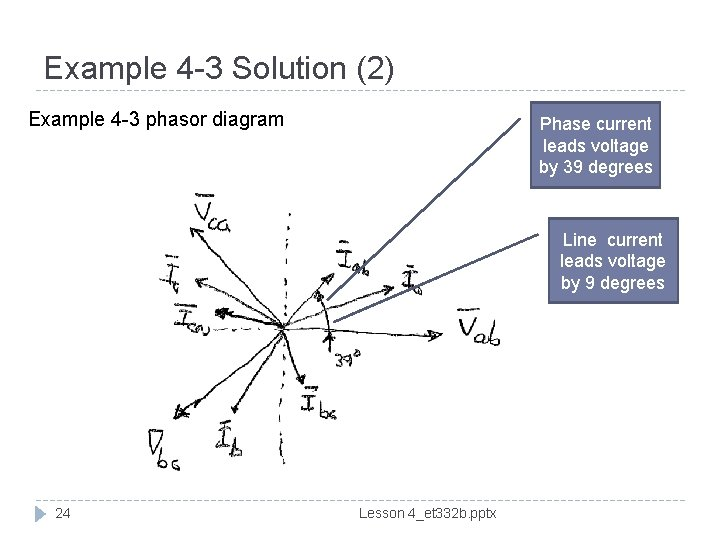Example 4 -3 Solution (2) Example 4 -3 phasor diagram Phase current leads voltage by 39 degrees Line current leads voltage by 9 degrees 24 Lesson 4_et 332 b. pptxEnd Lesson 4: Three Phase Sources and Loads ET 332 a Ac Motors, Generators and Power Systems 25 Lesson 4_et 332 b. pptx